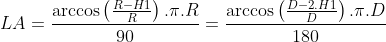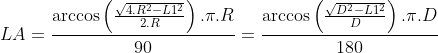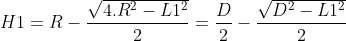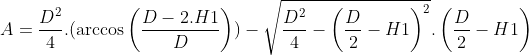Circle segment calculator, formulas, practical use.

Circle segment calculations

The circle segment calculator is designed for calculations of a circle radius and diameter of a circle segment, circumference and area of a circle segment, chord and arc length of a circle segment and height of a circle segment. For successful calculation, you need to know and enter into the calculator radius or diameter and at least one of other. Or chord length and circle segment height. Then press send or enter to run calculations.

Circle segment parameters sketch:Circle segment calculator form:

﻿
Unit system:
•

•

•

•

•

•

•

Circle segment calculator variables and constants explanation:

d – diameter
C – circumference
A – area
LA – arc length
π– 3,1415926535
H1– section height
L1 – chord length
– unspecified values
specified values
calculated values

The formula for circle arc length calculation:The formula for circle arc length calculation:The formula for circle chord length calculation:The formula for circle segment height calculation:The formula for circle segment area calculation:The formula for circle segment area calculation:The formula for circle segment circumference calculation:The formula for circle segment area calculation:Circle segment calculator use:

Calculations of circle segment for:

• technical calculation of D-shaped trimmed shaft in the engineering industry
• the cross-sectional area of a truncated cylinder, truncated tree trunk, pipe, tube, hose, rod, tunnel, pillar
• school calculations of a truncated cylinder